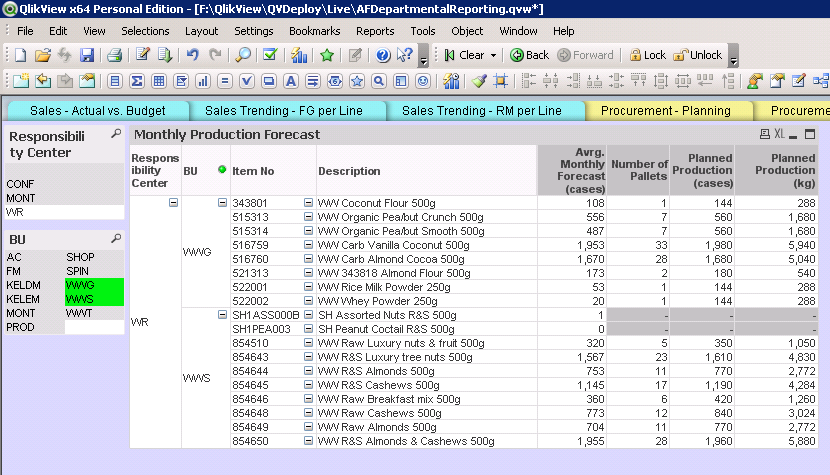# QlikView App Development

Discussion Board for collaboration related to QlikView App Development.

Announcements
Modernize Your QlikView Deployment webinar, Nov. 3rd. REGISTER
cancel
Showing results for
Did you mean:
HighlightedCreator

## Partial sum not working on pivot table

good day,

does anyone know why my partial sum on the pivot table does not want to show?1 Solution

Accepted Solutions
HighlightedMVP

I think all you might need is to surround your expression with Sum(Aggr(....., Dimensions))

http://help.qlik.com/en-US/qlikview/November2017/Subsystems/Client/Content/ChartFunctions/NestedAggr...

6 Replies
HighlightedMVP

What is your expression that you are using?

HighlightedSpecialist III

hello

maybe because one of the rows contains null in the value you are using

and the grouping function used returns null if one of the value is null

HighlightedSpecialist II

Hi,

can you add selection boxes on these fields to see if there is any data or not ?

HighlightedCreator

Hi Sunny,

my expressions are as follows (i have 4):

1. Average monthly forecast (cases):

if(IsNull

(sum ([Annual Sales])*-1/12 * (1 + ((sum([3 Months Sales]) - sum([Prior Year 3 Months Sales]))

/

sum([Prior Year 3 Months Sales])))

/

[FG Case weight])

,

(sum([3 Months Sales])*-1/3) / [FG Case weight]

,

(sum ([Annual Sales])*-1/12 * (1 + ((sum([3 Months Sales]) - sum([Prior Year 3 Months Sales]))

/

sum([Prior Year 3 Months Sales])))

/

[FG Case weight]) )

2. Number of pallets:

Ceil(

(if(IsNull

(sum ([Annual Sales])*-1/12 * (1 + ((sum([3 Months Sales]) - sum([Prior Year 3 Months Sales]))

/

sum([Prior Year 3 Months Sales])))

/

[FG Case weight])

,

(sum([3 Months Sales])*-1/3) / [FG Case weight]

,

(sum ([Annual Sales])*-1/12 * (1 + ((sum([3 Months Sales]) - sum([Prior Year 3 Months Sales]))

/

sum([Prior Year 3 Months Sales])))

/

[FG Case weight]) ) )

/

[FG Pallet Size]

)

3. Planned production (cases):

(Ceil(

(if(IsNull

(sum ([Annual Sales])*-1/12 * (1 + ((sum([3 Months Sales]) - sum([Prior Year 3 Months Sales]))

/

sum([Prior Year 3 Months Sales])))

/

[FG Case weight])

,

(sum([3 Months Sales])*-1/3) / [FG Case weight]

,

(sum ([Annual Sales])*-1/12 * (1 + ((sum([3 Months Sales]) - sum([Prior Year 3 Months Sales]))

/

sum([Prior Year 3 Months Sales])))

/

[FG Case weight]) ) )

/

[FG Pallet Size]

) )

* [FG Pallet Size]

4. Planned production (kg):

(Ceil(

(if(IsNull

(sum ([Annual Sales])*-1/12 * (1 + ((sum([3 Months Sales]) - sum([Prior Year 3 Months Sales]))

/

sum([Prior Year 3 Months Sales])))

/

[FG Case weight])

,

(sum([3 Months Sales])*-1/3) / [FG Case weight]

,

(sum ([Annual Sales])*-1/12 * (1 + ((sum([3 Months Sales]) - sum([Prior Year 3 Months Sales]))

/

sum([Prior Year 3 Months Sales])))

/

[FG Case weight]) ) )

/

[FG Pallet Size]

) )

* [FG Pallet Size]

*[FG Case weight]

HighlightedMVP

I think all you might need is to surround your expression with Sum(Aggr(....., Dimensions))

http://help.qlik.com/en-US/qlikview/November2017/Subsystems/Client/Content/ChartFunctions/NestedAggr...

HighlightedContributor

But I have created Dimensions using Valuelist and Still I don't see the SUM.